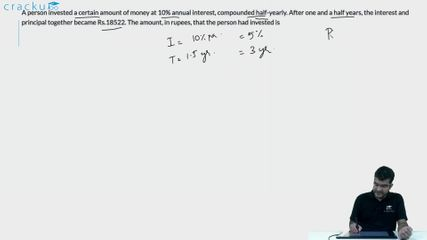Question 63

# A person invested a certain amount of money at 10% annual interest, compounded half-yearly. After one and a half years, the interest and principal together became Rs.18522. The amount, in rupees, that the person had invested is

Solution

Given,

Rate of interest = 10%

Since it is compounded half-yearly, R=5%

n=3

We know, A = $$P\left(1+\frac{R}{100}\right)^{^n}$$

18522 = $$P\left(1+0.05\right)^3$$

=> P = 16000

### View Video Solution